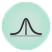# Mean Fuser¶Constructs relation matrices based on the average values of matrix elements.

## Signals¶

Inputs:

• Fusion Graph

A relational scheme of a data compendium.

• Relation

Relationships between two groups of objects.

Outputs:

• Mean-fitted fusion graph

Mean fuser.

• Relation

Relationships between two groups of objects.

## Description¶

The widget completes each relation matrix at the input based on the available data in the matrix. Unknown values in the matrix can be replaced with the values obtained by averaging matrix rows, matrix columns or the entire data matrix.1. Select the axis for mean value calculation:
• rows
• columns
• all
2. Output selected relation matrix, where unknown matrix elements are replaced with mean values.

## Example¶

Mean Fuser widget is useful for comparing RMSE values in Completion Scoring widget for the input data set. In the example below we have sampled movie ratings, fed the in-sample movie ratings data into Fusion Graph and from there into Completion Scoring for evaluation. We also fed the out-of-sample data from Matrix Sampler into Completion Scoring widget as out-of-sample movie ratings data is needed to assess how well the predicted values correspond to the true data. Finally, we compare prediction to those obtained by Mean Fuser.